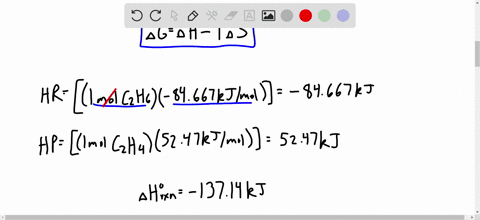Enroll in one of our FREE online STEM bootcamps. Join today and start acing your classes!View Bootcamps05:53
Problem 95

# Consider the following reaction:$$2 \mathrm{NOBr}(g) \rightleftharpoons 2 \mathrm{NO}(g)+\mathrm{Br}_{3}(g) \quad K=0.42 \mathrm{at} 373 \mathrm{K}$$Given that $S^{\circ}$ of $\operatorname{NOBr}(g)=272.6 \mathrm{J} / \mathrm{mol}$ . $\mathrm{K}$ and that $\Delta S_{\mathrm{rxn}}^{\circ}$ and $\Delta H_{\mathrm{rxn}}^{\circ}$ are constant with temperature, find(a) $\Delta S_{\mathrm{rxn}}^{\circ}$ at 298 $\mathrm{K}$(b) $\Delta G_{\mathrm{rxn}}^{\circ}$ at 373 $\mathrm{K}$(c) $\Delta H_{\mathrm{rxn}}^{\circ}$ at 373 $\mathrm{K}$(d) $\Delta H_{\mathrm{f}}^{\circ}$ of $\mathrm{NOBr}$ at 298 $\mathrm{K}$(e) $\Delta G_{\text { rxn }}^{\circ}$ at 298 $\mathrm{K}$(f) $\Delta G_{\mathrm{f}}^{\circ}$ of $\mathrm{NOBr}$ at 298 $\mathrm{K}$

Check back soon!

## Discussion

You must be signed in to discuss.

## Video Transcript

So for the reaction, we can get the entropy change for the reaction for the system from these standard interviews in the, uh, appendix three in the back of the book. And so we would take twice the value for water and subtract from it twice the value for hydrogen, plus the value for oxygen. And, uh, having done that, I gotta minus 3 27 jewels for Kellen. And then we can calculate the delta s of the surroundings from the delta h of the system and the temperature. And so we got a pretty large negative because what? We're going from three moles of gas here to two moles of liquid. So yeah, big negative entropy change for the system, but it gives off a huge amount of heat. And so a large positive in tribute change for the surroundings. And of course, we know that hydrogen burns explosively and eso for the tell us of the universe combining Ah, the reaction and thes surroundings. We get a very large positive, uh, entropy change

#### You're viewing a similar answer. To request the exact answer, fill out the form below:

Our educator team will work on creating an answer for you in the next 6 hours.